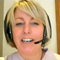Study Notes

# Calculating Breakeven Output - Formulae

Level:
AS
Board:
AQA, Edexcel, OCR, IB

Last updated 22 Mar 2021

Let's look at the most common way of calculating breakeven output - using formulae

Here is a table showing the sales, variable costs, fixed costs and profits from various levels of output for a one-product business:

The product is sold for £10 per unit. The variable cost per unit is £4. Fixed costs are £40,000 (the same at each level of output).

Let's use the same information as above to show how a formula can be used to quickly calculate the break-even output.

Remember contribution per unit? Here is a reminder of this crucial and really useful formula:

Contribution per unit = selling price per unit less variable cost per unit

In our example, contribution per unit = £10 less £4 = £6 per unit

Now apply the classic formula for calculating breakeven output:

Break-even output (units) = Fixed costs (£) / Contribution per unit (£)

So, break-even output = £40,000 divided by £6 = 6,666 units

Note: break-even output is always expressed in terms of units

So break-even output = 6,666 units

If the information is available, it is always quicker and easier to use this formula rather than use a table or draw a chart.

12th June 2018

### Location issues - offshoring out of China?

31st May 2018### Lunchtime Learning with the Calculation Practice Book!

2nd February 2018

### Waste not, want not

5th December 2017

### Flying first class?

15th November 2017### AQA A Level Business Linear Worksheets

21st September 2017### New Edexcel A Level Business (Year 1) Numerical Assessment

13th September 2017### Introducing Topic Plus PowerPoints for Edxcel GCSE Business (9-1) - Theme 1

11th September 2017

© 2002-2022 Tutor2u Limited. Company Reg no: 04489574. VAT reg no 816865400.